In the circuit shown, a meter bridge is in its balanced state. The meter bridge wire has a resistance 0.1 ohm/cm. The value of unknown resistance X and the current drawn from the battery of negligible resistance is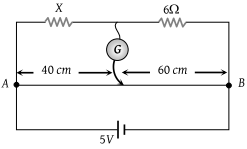(1) 6 Ω, 5 amp

(2) 10 Ω, 0.1 amp

(3) 4 Ω, 1.0 amp

(4) 12 Ω, 0.5 amp

Concept Questions :-

Meter bridge and potentiometer
High Yielding Test Series + Question Bank - NEET 2020

Difficulty Level:

AB is a potentiometer wire of length 100 cm and its resistance is 10 ohms. It is connected in series with a resistance R = 40 ohms and a battery of e.m.f. 2 V and negligible internal resistance. If a source of unknown e.m.f. E is balanced by 40 cm length of the potentiometer wire, the value of E is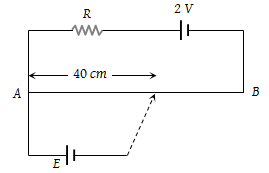(1) 0.8 V

(2) 1.6 V

(3) 0.08 V

(4) 0.16 V

Concept Questions :-

Meter bridge and potentiometer
High Yielding Test Series + Question Bank - NEET 2020

Difficulty Level:

The measurement of the voltmeter in the following circuit is :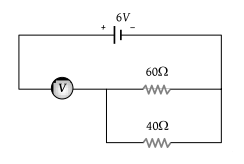(1) 2.4 V

(2) 3.4 V

(3) 4.0 V

(4) 6.0 V

Concept Questions :-

Kirchoff's voltage law
High Yielding Test Series + Question Bank - NEET 2020

Difficulty Level:

The potential difference across the 100Ω resistance in the following circuit is measured by a voltmeter of 900 Ω resistance. The percentage error made in reading the potential difference is :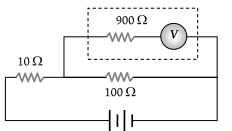(1) $\frac{10}{9}$

(2) 0.1

(3) 1.0

(4) 10.0

Concept Questions :-

Combination of resistors
High Yielding Test Series + Question Bank - NEET 2020

Difficulty Level:

If the ammeter in the given circuit reads 2 A, the resistance R is :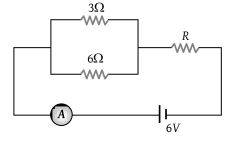(1) 1 ohm

(2) 2 ohm

(3) 3 ohm

(4) 4 ohm

Concept Questions :-

Kirchoff's voltage law
High Yielding Test Series + Question Bank - NEET 2020

Difficulty Level:

Two resistances of 400 Ω and 800 Ω are connected in series with a 6-volt battery of negligible internal resistance. A voltmeter of resistance 10,000 Ω is used to measure the potential difference across 400 Ω. The error in the measurement of potential difference in volts approximately is :

(1) 0.01

(2) 0.02

(3) 0.03

(4) 0.05

Concept Questions :-

Kirchoff's voltage law
High Yielding Test Series + Question Bank - NEET 2020

Difficulty Level:

The length of a wire of a potentiometer is 100 cm, and the emf of its standard cell is E volt. It is employed to measure the e.m.f of a battery whose internal resistance is 0.5 Ω. If the balance point is obtained at l = 30 cm from the positive end, the e.m.f. of the battery is :

(1) $\frac{30E}{100}$

(2) $\frac{30E}{100.5}$

(3) $\frac{30E}{\left(100-0.5\right)}$

(4) $\frac{30\left(E-0.5i\right)}{100}$, where i is the current in the potentiometer

Concept Questions :-

Grouping of cells
High Yielding Test Series + Question Bank - NEET 2020

Difficulty Level:

What is the reading of the voltmeter in the following figure?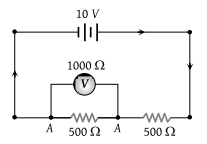(1) 3 V

(2) 2 V

(3) 5 V

(4) 4 V

Concept Questions :-

Kirchoff's voltage law
High Yielding Test Series + Question Bank - NEET 2020

Difficulty Level:

The current flowing in a coil of resistance 90 Ω is to be reduced by 90%. What value of resistance should be connected in parallel with it

(1) 9 Ω

(2) 90 Ω

(3) 1000 Ω

(4) 10 Ω

High Yielding Test Series + Question Bank - NEET 2020

Difficulty Level:

A galvanometer of 50 ohm resistance has 25 divisions. A current of 4 × 10–4 ampere gives a deflection of one division. To convert this galvanometer into a voltmeter having a range of 25 volts, it should be connected with a resistance of :

(1) 2500 Ω as a shunt

(2) 2450 Ω as a shunt

(3) 2550 Ω in series

(4) 2450 Ω in series

Concept Questions :-

Meter bridge and potentiometer